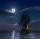# Density + reason - math problems

#### Number of problems found: 7

• Force meterWe put the statuette on the force meter. The force meter showed a value 25 N. Then we placed the statuette on the force meter completely immersed in the water. The force meter showed a value 17 N. What is the volume of the statuette?
• FreezerThe freezer has the shape of a cuboid with internal dimensions of 12 cm, 10 cm, 30 cm. A layer of ice of 23 mm thick was formed on the inner walls (and on the opening) of the freezer. How many liters of water will drain if we dispose the freezer?
• Sea waterSeawater contains about 4.3% salt. How many dm3 of distilled water we must pour into 5 dm3 of sea water to get water with 1.8% salt?
• Sea on the MoonAssume that the Moon has sea, the same composition as on the Earth (has the same density of saltwater). Calculate dive of the boat floating in the sea on the Moon, when on Earth has dive 3.6 m. Consider that the Moon has 6.5-times smaller gravitational ac
• PumpWhat power has a pump output to move 4853 hl of water to a height of 31 m for 8 hours?
• Cu thiefThe thief stole 121 meters copper wire with a cross-section area of 103 mm2. Calculate how much money gets in the scrap redemption if redeemed copper for 4.6 Eur/kg? The density of copper is 8.96 t/m3.
• Eiffel TowerEiffel Tower in Paris is 300 meters high and is made of steel. Its weight is 8000 tons. How tall is the tower model made ​​of the same material if it weighs is 2.4 kg?

We apologize, but in this category are not a lot of examples.
Do you have an exciting math question or word problem that you can't solve? Ask a question or post a math problem, and we can try to solve it.

We will send a solution to your e-mail address. Solved examples are also published here. Please enter the e-mail correctly and check whether you don't have a full mailbox.

Tip: Our Density units converter will help you with the conversion of density units. Density - math problems. Reason - math problems.